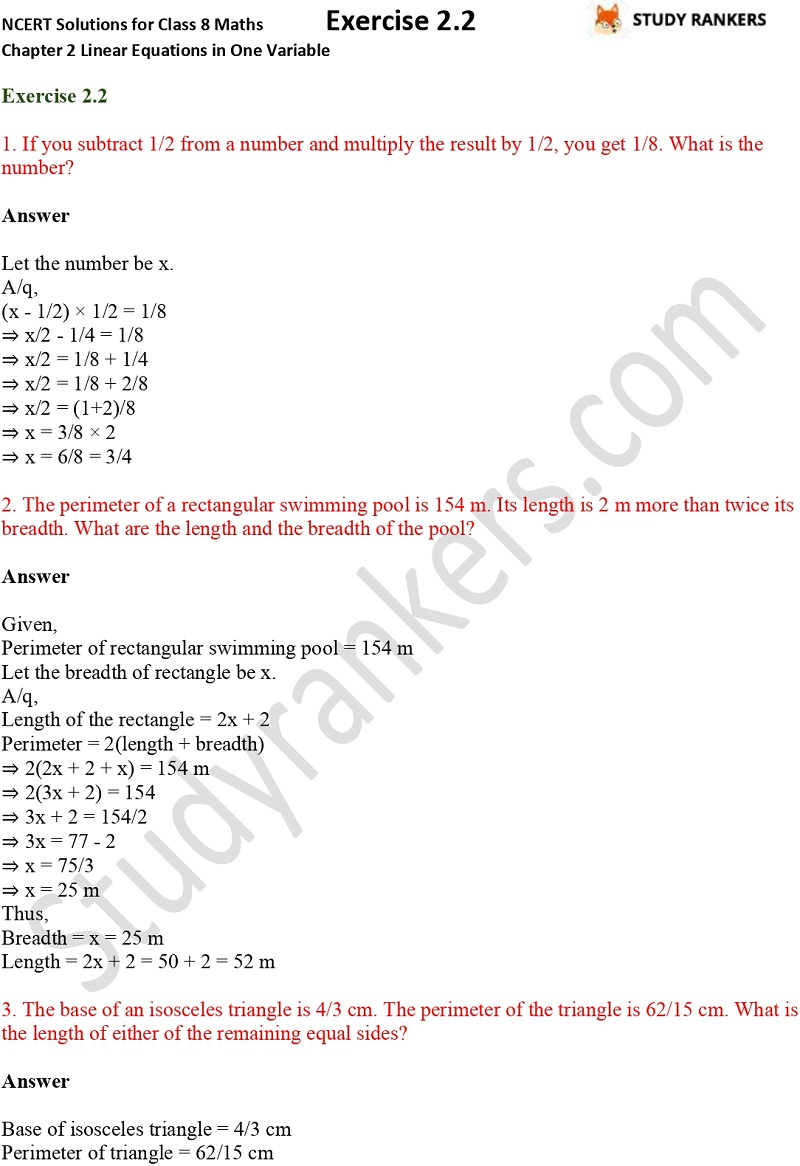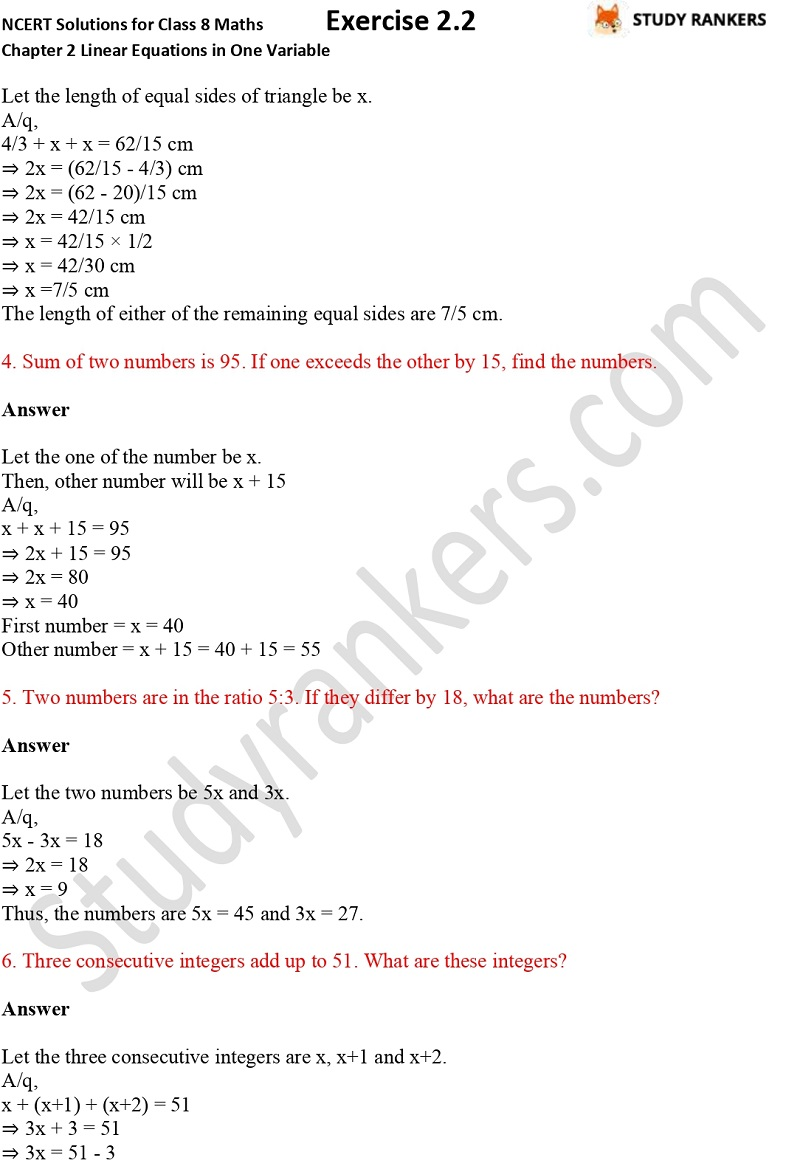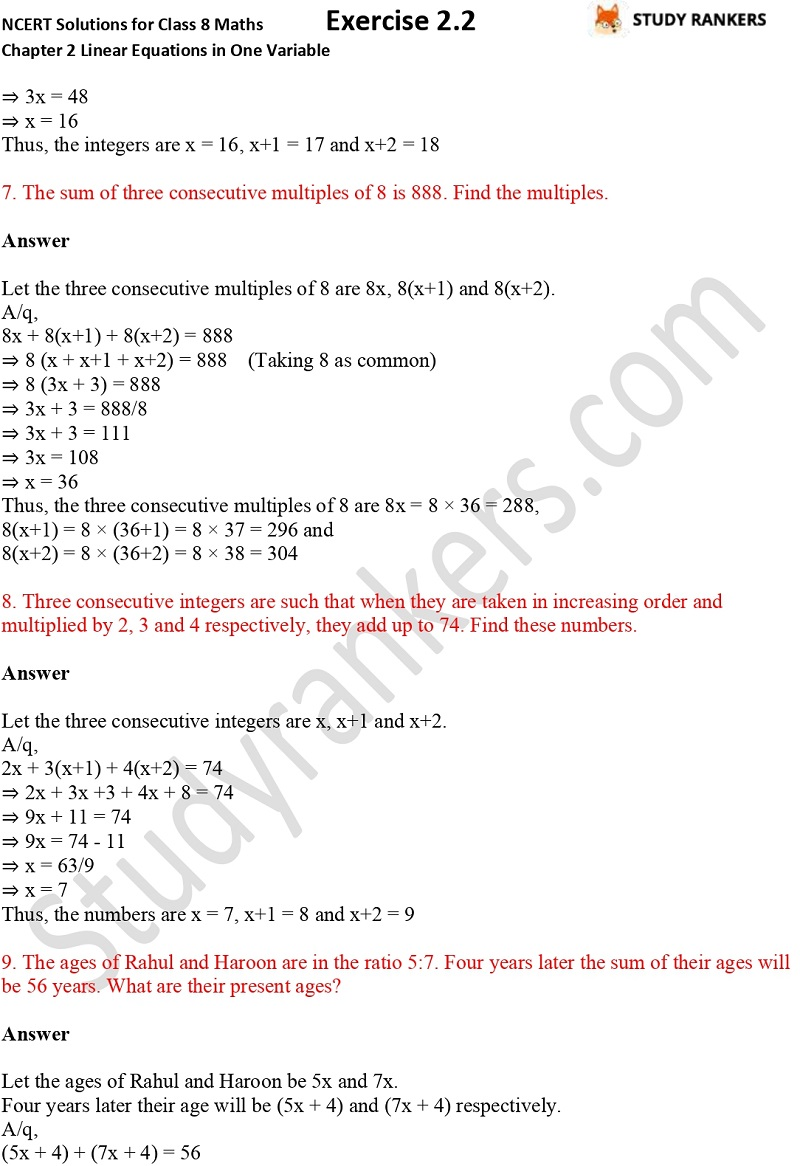>

## NCERT Solutions for Class 8 Maths Chapter 2 Linear Equations in One Variable Exercise 2.2

If you're finding NCERT Solutions of Chapter 2 Linear Equations in One Variable Exercise 2.2 Class 8 Maths then you can get them here. NCERT Solutions for Class 8 Maths is provided here that will help you in completing homework. These NCERT questions and answers are updated as per the latest syllabus and are detailed and accurate so you can solve your doubts if you have any while solving. It will help you a lot in improving your marks in the examinations.

Exercise 2.2 has total 16 questions in the exercise which are about finding the number of winners, finding age of persons etc.X